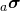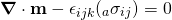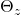# INTERNAL POLAR CONTINUUM THEORIES FOR SOLID AND FLUENT CONTINUA

Michael Powell, J. N. Reddy, and K. S. Surana

## Objective

In Lagrangian description of solid matter, displacements and displacement gradients contain all of the information needed to describe the deformation of a solid continua. Similarly, for fluent continua, the velocities and velocity gradients contain the complete physics of deformation. The measures of deformation used in classical continuum mechanics only consider parts of the displacement and velocity gradient tensors responsible for pure stretch and pure stretch rates, ignoring rotations and rates of rotations. This is a great benefit, as it helps to guarantee that the resulting mathematical framework does not change with rotation of the coordinate system.

We can observe however, that polar decomposition of the displacement (or velocity) gradient tensor at neighboring material points may result in varying rotations (or rates of rotations) from point to point. That is, part of the rotation (or rate of rotation) tensor describes the physics of rigid body rotation, and part of it describes some physics that is not rigid body rotation. These varying rotations, if resisted by the continua, will result in varying internal moments which provide a mechanism for additional energy storage or dissipation. In this work, we seek to derive a continuum thermodynamic framework which incorporates this additional physics due to varying rotations between material points. Since the resulting theories include effects from varying internal rotations without introducing any external mechanism (stress couples, micro-rotations, etc.) we call these theories “Internal polar continuum theories” for solid and fluent continua.

## Background

The concept of stress couples was introduced by Voigt in 1881 by assuming a couple or moment per unit area on the oblique plane of a deformed tetrahedron in addition to the stress or force per unit area. The Cosserat brothers  provided the first comprehensive treatment of a polar continuum, by introducing a theory which includes 6 degrees of freedom at each material point, 3 displacements and 3 rotations. Truesdell , Toupin  and many others have explored this and similar theories. In 1964, Eringen  introduced a general theory for so-called micromorphic continua, which includes micro-stretch as well as micro-rotation. Yang et. al.  present a modified couple stress based theory, including a balance law for moments of moments (or couples) to establish the symmetry of the couple stress tensor. More recently Srinivasa and Reddy , and Arbind et. al.  have derived elasticity theories based on rotation gradient dependent strain energy, and provide results for non-linear beams and plates. It should be noted that the aforementioned theories rely on the introduction of additional degrees of freedom (in the case of micro-polar theories) or a length scale parameter (in the case of stress couple theories), and therefore are not the same as the theory that is considered here.

## Technical Approach

For the complete details of the derivation, see references [8-11], however here we highlight the differences between the proposed continuum theory for the case of linear elastic solid matter and the classical theory of elasticity.

Balance of linear momenta:whereis the symmetric part of the Cauchy stress tensor andis the anti-symmetric part.

Balance of angular momenta:whereis the Cauchy moment tensor, which results from the continuum resisting varying internal rotations. In classical continuum mechanics, the balance of angular momenta is used to establish symmetry of the Cauchy stress tensor, however here it relates the antisymmetric part of the Cauchy stress tensor to the gradients of the moment tensor.

Balance of moments of moments (or couples):In order to satisfy this, the moment tensormust be symmetric.

These balance laws, along with the first and second laws of thermodynamics, and constitutive theories for the symmetric part of the stress tensor, moment tensor, and heat vector form a complete mathematical model for solid continua in Lagrangian description. Details of this model and a similar one for fluent continua can be found in references [8-11].

## Numerical Examples

Consider a thin plate with lengthof 20 inches, breadthof 0.5 inches and thicknessof 0.1 inches. Withinches the dimensionless plate is. We consider loads applied in the plane of the plate. We choosepsi, hence dimensionless. The dimensionless value of the polar modulusis increased starting with 0.0 and is chosen to be a fraction of the dimensionless modulus of elasticity (which is unity). Clearly for, the internal polar physics is absent i.e. the usual small strain approximation theory of elasticity applies for this case.

The following figure shows the schematic representation and boundary conditions of the plate considered here. Vertical displacement is constrained at points A and B, and horizontal displacement is constrained at, the left and right boundaries are traction free, and the top and bottom boundaries have a vertical force applied in the negativedirection. Numeric results are obtained using the least-squares finite element method (LSFEM) [12-14], and the integrated sum of squares of the residuals of the results presented here are.Schematic and boundary conditions for simply-supported plate.

Plots of the vertical displacementand rotationshow that by increasing the effect of internal polar physics (by increasing the value ofresults in lower magnitudes of displacement. This is due to the additional mechanism for energy storage provided by the internal polar physics.Vertical displacement at y = 0.025.z-component of rotation tensor at y = 0.025.

We can see that as the effect of polar physics increases, the symmetric part of the shear stress decreases and the anti-symmetric part becomes more dominant. The anti-symmetric part of the shear stress is of course balanced by the moment tensor, which increases in magnitude asincreases.Moment tensor at y = 0.025.Symmetric part of the shear component of the Cauchy stress tensor at y = 0.025.Anti-symmetric part of the shear component of the Cauchy stress tensor at y = 0.025.

## Conclusions

The development of the polar theory proposed here for isotropic, homogeneous solid and fluent continua is motivated by the fact that the polar decomposition of changing displacement and velocity gradient tensors at a location and its neighbors can result in different rotations and rates of rotations. If these are resisted by the continua there will result conjugate internal moments. These conjugate internal rotations and rates of rotations and the internal moments can result in additional energy storage and dissipation. The currently used thermodynamic framework for isotropic, homogeneous solid and fluent continua completely ignores this physics in the derivation of the conservation and balance laws. The theory resulting from the new physics considered here is in fact ‘an internal polar theory’ as it considers internal rotations and rates of rotations and moments as conjugate pairs.

## References

 Cosserat, E. and Cosserat, F., 1909. Théorie des corps déformables. Paris, 3, pp.17-29.
 Ericksen, J.L. and Truesdell, C., 1957. Exact theory of stress and strain in rods and shells. Archive for Rational Mechanics and Analysis, 1(1), pp.295-323.
 Toupin, R.A., 1964. Theories of elasticity with couple-stress. Archive for Rational Mechanics and Analysis, 17(2), pp.85-112.
 Eringen, A.C. and Suhubi, E.S., 1964. Nonlinear theory of simple micro-elastic solids—I. International Journal of Engineering Science, 2(2), pp.189-203.
 Yang, F.A.C.M., Chong, A.C.M., Lam, D.C.C. and Tong, P., 2002. Couple stress based strain gradient theory for elasticity. International Journal of Solids and Structures, 39(10), pp.2731-2743.
 Srinivasa, A.R. and Reddy, J.N., 2013. A model for a constrained, finitely deforming, elastic solid with rotation gradient dependent strain energy, and its specialization to von Karman plates and beams. Journal of the Mechanics and Physics of Solids, 61(3), pp.873-885.
 Reddy, J.N. and Arbind, A., 2012. Bending relationships between the modified couple stress-based functionally graded Timoshenko beams and homogeneous Bernoulli–Euler beams. Annals of Solid and Structural Mechanics, 3(1-2), pp.15-26.
 Surana, K.S, Powell, M. and Reddy, J.N., 2015. A more complete thermodynamic framework for solid continua. Journal of Thermal Engineering, 1(6), pp.446-459.
 Surana, K.S., Powell, M. and Reddy, J.N., 2015. A more complete thermodynamic framework for fluent continua. Journal of Thermal Engineering, 1(6).
 Surana, K.S., Powell, M. and Reddy, J.N., 2015. Constitutive theories for internal polar thermoelastic solid continua. Journal of Pure and Applied Mathematics: Advances and applications, 14(2), pp.89-150.
 Surana, K.S., Powell M., and Reddy J.N., 2015. Ordered Rate Constitutive Theories for Internal Polar Thermofluids. International J. of Math. Sci. & Engg. Appls. (IJMSEA) 9(3) pp.51-67
 Surana, K.S., Ahmadi, A.R. and Reddy, J.N., 2002. The k-version of finite element method for self-adjoint operators in BVP. International Journal of Computational Engineering Science, 3(02), pp.155-218.
 Surana, K.S., Ahmadi, A.R. and Reddy, J.N., 2003. The k-version of finite element method for non-self-adjoint operators in BVP. International journal of computational engineering science, 4(04), pp.737-812.
 Surana, K.S., Ahmadi, A.R. and Reddy, J.N., 2004. The k-version of finite element method for nonlinear operators in BVP. International journal of computational engineering science, 5(01), pp.133-207.